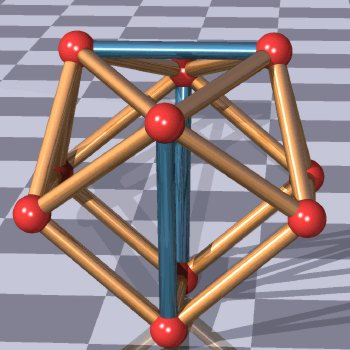Distant points on a supersphere in n-dimensional space

How to find m points on a supersphere x12 + x22 + ... + xn2 = 1 in n-dimensional space such that the minimum distance between any two of these points is as large as possible?

I first tried the following pascal program:

program maxmin;
label 1;
var i,j,i1,i2,m,n,a,b:integer; max,min,som,s:real; p:array[1..20,1..8] of real;
begin
writeln('aantal punten? (m, max 20)'); readln(m);
max:=0;
while true do
begin
1: min:=100;
for j:=1 to n-1 do p[1,j]:=0; p[1,n]:=1;
for i:=2 to m do
begin
repeat
som:=0;
for j:=1 to n-1 do
begin
p[i,j]:=2*random-1;
som:=som+p[i,j]*p[i,j]
end
until (som<=1);
p[i,n]:=sqrt(1-som); if random>0.5 then p[i,n]:=-p[i,n];
end;
for i1:=1 to m do for i2:=i1+1 to m do
begin
s:=0;
for j:=1 to n do s:=s+(p[i1,j]-p[i2,j])*(p[i1,j]-p[i2,j]);
if s<=min then begin a:=i1; b:=i2; min:=s end;
if min<=max then goto 1;
end;
max:=min; writeln;
writeln(sqrt(max):13:10);
writeln(a:3,b:3);
for i:=1 to m do
begin
for j:=1 to n do write(p[i,j]:8:5); writeln
end; writeln
end
end.

For instance, this program gives a configuration of 10 points on a sphere in 3-dimensional space such that (roughly) the minimal distance between any two of them (0.9524) is 87% of the theoretical maximum (1.0914) within a few hours.
Next, by varying the coordinates of the two closest points in this configuration with steps of 0.01, and repeating this procedure, we get a new configuration of 10 points on the sphere such that (roughly) the minimal distance between any two of them (0.9778) is 90% of the theoretical maximum (1.0914).
Next, fixing the coordinates of six points in this configuration at (0,0,1) and (cos(θ)cos(2kπ/5),cos(θ)sin(2kπ/5),sin(θ)) with equal mutual distances, and choosing the other four points at random like above, we get a new configuration of 10 points on the sphere such that (roughly) the minimal distance between any two of them (1.025) is 94% of the theoretical maximum (1.0914).
Next, by varying the coordinates of the two closest points in this configuration with steps of 0.001, and repeating this procedure, we get a new configuration of 10 points on the sphere such that (roughly) the minimal distance between any two of them (1.0258) is 94% of the theoretical maximum (1.0914).

This configuration is (roughly) the following:

0.00000 0.00000 1.00000
0.89443 0.00000 0.44721
0.27639 0.85065 0.44721
-0.72361 0.52573 0.44721
-0.72361 -0.52573 0.44721
0.27639 -0.85065 0.44721
-0.78118 -0.20289 -0.59042
-0.26384 0.70751 -0.65561
0.80143 0.24997 -0.54335
0.32744 -0.73356 -0.59554

We can find coordinates for the ten points in Danzer's optimal configuration as follows:O1(0,-cos(θ1),-sin(θ1))
O2(0,cos(θ1),-sin(θ1))
T1(cos(α)cos(θ2),-sin(α)cos(θ2),-sin(θ2))
T2(cos(α)cos(θ2),sin(α)cos(θ2),-sin(θ2))
T3(-cos(α)cos(θ2),sin(α)cos(θ2),-sin(θ2))
T4(-cos(α)cos(θ2),-sin(α)cos(θ2),-sin(θ2))
U1(0,-cos(θ3),sin(θ3))
U2(0,cos(θ3),sin(θ3))
V1(cos(θ4),0,sin(θ4))
V2(-cos(θ4),0,sin(θ4))

with θ1 and θ4 'about' π/3, with θ2 and θ3 'about' π/6, and with α 'about' π/4.

By requiring O1O2 = O1T1 = T1T2 = T1U1 = U1V1 and O1U1 = V1V2, we find in degrees rounded to 2 decimals after the decimal point

α = 33.63, θ1 = 56.61, θ2 = 6.28, θ3 = 34.15, θ4 = 44.62 .